# Excel substring: how to extract text from cell

The tutorial shows how to use the Substring functions in Excel to extract text from a cell, get a substring before or after a specified character, find cells containing part of a string, and more.

Before we start discussing different techniques to manipulate substrings in Excel, let's just take a moment to define the term so that we can begin on the same page. So, what is a substring? Simply, it's part of a text entry. For example, if you type something like "AA-111" in a cell, you'd call it an alphanumeric string, and any part of the string, say "AA", would be a substring.

Although there is no such thing as Substring function in Excel, there exist three Text functions (LEFT, RIGHT, and MID) to extract a substring of a given length. Also, there are FIND and SEARCH functions to get a substring before or after a specific character. And, there are a handful of other functions to perform more complex operations such as extracting numbers from a string, replacing one substring with another, looking up partial text match, etc. Below you will find formula examples to do all this and a lot more.

## How to extract substring of a certain length

Microsoft Excel provides three different functions to extract text of a specified length from a cell. Depending on where you want to start extraction, use one of these formulas:

• LEFT function - to extract a substring from the left.
• RIGHT function - to extract text from the right.
• MID function - to extract a substring from the middle of a text string, starting at the point you specify.

As is the case with other formulas, Excel substring functions are best to learn from an example, so let's look at a few ones.

### Extract substring from start of string (LEFT)

To extract text from the left of a string, you use the Excel LEFT function:

LEFT(text, [num_chars])

Where text is the address of the cell containing the source string, and num_chars is the number of characters you want to extract.

For example, to get the first 4 characters from the beginning of a text string, use this formula:

`=LEFT(A2,4)`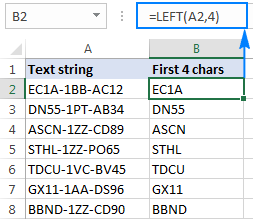### Get substring from end of string (RIGHT)

To get a substring from the right part of a text string, go with the Excel RIGHT function:

RIGHT(text, [num_chars])

For instance, to get the last 4 characters from the end of a string, use this formula:

`=RIGHT(A2,4)`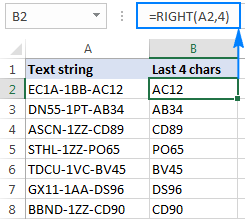### Extract text from middle of string (MID)

If you are looking to extract a substring starting in the middle of a string, at the position you specify, then MID is the function you can rely on.

Compared to the other two Text functions, MID has a slightly different syntax:

MID(text, start_num, num_chars)

Aside from text (the original text string) and num_chars (the number of characters to extract), you also indicate start_num (the starting point).

In our sample data set, to get three characters from the middle of a string beginning with the 6th character, you use the following formula:

`=MID(A2,6,3)`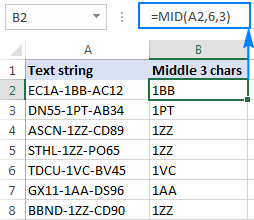Tip. The output of the Right, Left and Mid formulas is always text, even when you are extracting a number from a text string. If you want to operate on the result as a number, then wrap your formula in the VALUE function like this: `=VALUE(MID(A2,6,3))`

## Extract substring before or after a given character

As shown in the above examples, the Left, Right and Mid functions cope nicely with uniform strings. When you are dealing with text strings of variable length, more complex manipulations shall be needed.

Note. In all of the below examples, we will be using the case-insensitive SEARCH function to get the position of a character. If you want a case-sensitive formula, use the FIND function instead.

### How to extract text before a specific character

To get a substring preceding a given character, two things are to be done: first, you determine the position of the character of interest, and then you pull all characters before it. More precisely, you use the SEARCH function to find the position of the character, and subtract 1 from the result, because you don't want to include the character itself in the output. And then, you send the returned number directly to the num_chars argument of the LEFT function:

LEFT(cell, SEARCH("char", cell)-1)

For example, to extract a substring before the hyphen character (-) from cell A2, use this formula:

`=LEFT(A2, SEARCH("-",A2)-1)`

No matter how many characters your Excel string contains, the formula only extracts text before the first hyphen: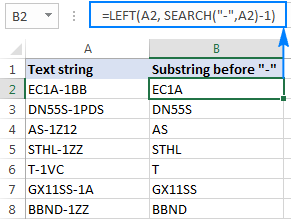### How to extract text after character

To get text following a specific character, you use a slightly different approach: get the position of the character with either SEARCH or FIND, subtract that number from the total string length returned by the LEN function, and extract that many characters from the end of the string.

RIGHT(cell,LEN(cell)-SEARCH("char", cell))

In our example, we'd use the following formula to extract a substring after the first hyphen:

`=RIGHT(A2,LEN(A2)-SEARCH("-",A2))`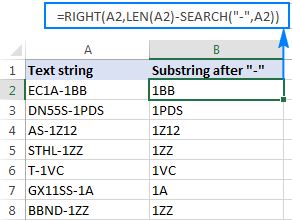### How to extract text between two instances of a character

To get a substring between two occurrences of a certain character, use the following generic formula:

MID(cell, SEARCH("char", cell)+1, SEARCH ("char", cell, SEARCH ("char", cell)+1) - SEARCH ("char", cell)-1)

The first two arguments of this MID formula are crystal clear:

Text is the cell containing the original text string.

Start_num (starting point) - a simple SEARCH formula returns the position of the desired character, to which you add 1 because you want to start extraction with the next character.

Num_chars (number of chars to extract) is the trickiest part:

• First, you work out the position of the second occurrence of the character by nesting one Search function within another.
• After that, you subtract the position of the 1st occurrence from the position of the 2nd occurrence, and subtract 1 from the result since you don't want to include the delimiter character in the resulting substring.

For example, to extract text surrounded by two hyphens, you'd use this formula:

`=MID(A2, SEARCH("-",A2) + 1, SEARCH("-",A2,SEARCH("-",A2)+1) - SEARCH("-",A2) - 1)`

The screenshot below shows the result: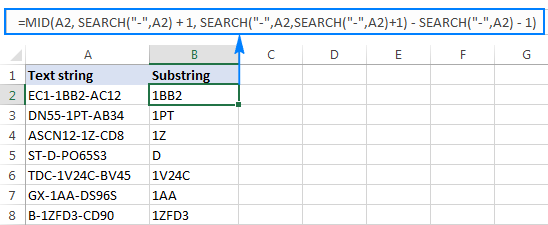If you are looking to extract text between 2nd and 3rd or 3nd and 4th occurrences of the same character, you can use a more compact SEARCH SUBSTITUTE combination to get the character's position, as explained in How to find Nth occurrence of a character in a string:

FIND(CHAR(1),SUBSTITUTE(cell,character,CHAR(1),Nth occurrence))

In our case, we could extract a substring between the 2nd and 3rd hyphens with the following formula:

`=MID(A2, FIND(CHAR(1),SUBSTITUTE(A2,"-",CHAR(1),2))+1, FIND(CHAR(1),SUBSTITUTE(A2,"-",CHAR(1),3)) - FIND(CHAR(1),SUBSTITUTE(A2,"-",CHAR(1),2))-1)`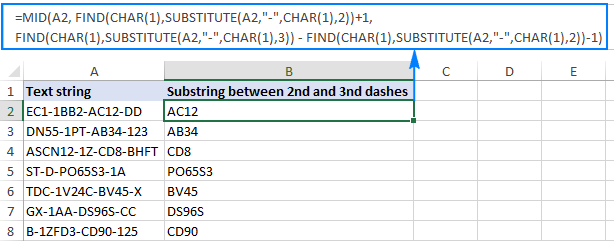## How to find substring in Excel

In situations when you don't want to extract a substring and only want to find cells containing it, you use the SEARCH or FIND function as shown in the above examples, but perform the search within the ISNUMBER function. If a cell contains the substring, the Search function returns the position of the first character, and as long as ISNUMBER gets any number, it returns TRUE. If the substring is not found, the search results in an error, forcing ISNUMBER to return FALSE.

ISNUMBER(SEARCH("substring", cell))

Supposing, you have a list of British postcodes in column A and you want to find those that contain the substring "1ZZ". To have it done, use this formula:

`=ISNUMBER(SEARCH("1zz", A2))`

The results will look something similar to this: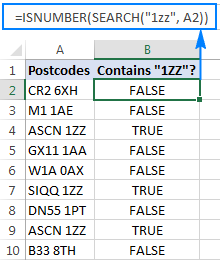If you'd like to return your own message instead of the logical values of TRUE and FALSE, nest the above formula into the IF function:

`=IF(ISNUMBER(SEARCH("1zz", A2)), "Yes", "")`

If a cell contains the substring, the formula returns "Yes", an empty string ("") otherwise: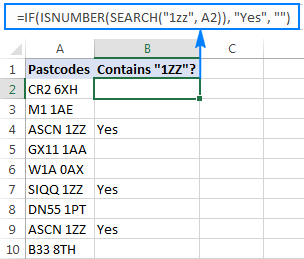As you may remember, the Excel SEARCH function is case-insensitive, so you use it when the character case does not matter. To get your formula to distinguish the uppercase and lowercase characters, opt for the case-sensitive FIND function.

For more information on how to find text and numbers in Excel, please see If cell contains formula examples.

## How to extract text from cell with Ultimate Suite for Excel

As you have just seen, Microsoft Excel provides an array of different functions to work with text strings. In case you are unsure which function is best suited for your needs, commit the job to our Ultimate Suite for Excel. With these tools in your Excel's arsenal, you just go to Ablebits Data tab > Text group, and click Extract: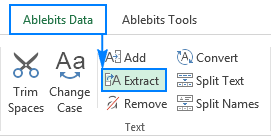Now, you select the source cells, and whatever complex strings they contain, a substring extraction boils down to these two simple actions:

1. Specify how many characters you want to get from the start, end or middle of the string; or choose to extract all text before or after a given character.
2. Click Insert Results. Done!

For example, to pull the domain names from the list of email addresses, you select the All after text radio button and type @ in the box next to it. To extract the user names, you select the All before text radio button, as shown in the screenshot below.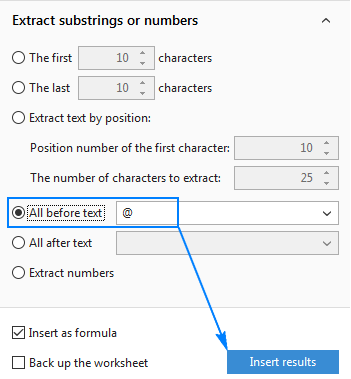And you will get the following results in a moment: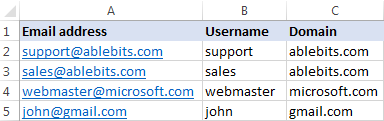Apart from speed and simplicity, the Extract Text tool has extra value - it will help you learn Excel formulas in general and substring functions in particular. How? By selecting the Insert as formula checkbox at the bottom of the pane, you ensure that the results are output as formulas, not values.

In this example, if you select cells B2 and C2, you will see the following formulas, respectively:

`=IFERROR(LEFT(A2,SEARCH("@",A2)-1),"")`

• To extract domain:

`=IFERROR(RIGHT(A2, LEN(A2)- SEARCH("@",A2) - LEN("@") + 1),"")`

How much time would it take you to figure out these formulas on your own? ;)

Since the results are formulas, the extracted substrings will update automatically as soon as any changes are made to the original strings. When new entries are added to your data set, you can copy the formulas to other cells as usual, without having to run the Extract Text tool anew.

If you are curious to try this as well as many other useful features included with Ultimate Suite for Excel, you are welcome to download evaluation version.

## More formulas for substrings in Excel

In this tutorial, we have demonstrated some classic Excel formulas to extract text from string. As you understand, there can be almost infinite variations of these basic scenarios. Below you will find a few more formula examples where the Text functions come in handy.War in Ukraine. Here's what Ablebits is doing to make sure our team and projects are safe.